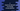# C program to insert an element in an array at any specific position# C program to insert an element in an array at any specific position :

In this C programming tutorial, we will learn how to insert an element in an array at any user-provided specific position. The array elements will be entered by the user. The program will print out the final array after the insertion is completed. For example, if our original array is [1,2,3,4] and if one new value 5 is inserted on the third position, the program will print out [1,2,5,3,4] as the output.

### The algorithm to use :

In this program, we will use the below algorithm :

1. The program will create one empty array with size 100. We are assuming that the array will not exceed this size.
2. It will then ask the user to enter the array elements one by one.
3. Each element will be stored in the array starting from index 0 .
4. Ask the user to provide the position and new element to enter to the array.
5. Starting from the position, move all elements of the array to right by one step.
6. This new adjustment will allow us to put the new element on the user provided position.
7. Finally, print out the array to the user.

### C program :

Let’s try to implement the above steps :

``````//1
#include <stdio.h>
#define MAX_ARRAY_SIZE 100

int main()
{
//2
int size;
int newNumber, position;
int i;
int numArray[MAX_ARRAY_SIZE];

//3
printf("Enter size of the array : ");
scanf("%d", &size);

//4
for (i = 0; i < size; i++)
{
printf("Enter value for position %d : ", i);
scanf("%d", &numArray[i]);
}

//5
printf("Enter the number you want to add to this array : ");
scanf("%d", &newNumber);

//6
printf("Enter the position in the array to add this number : ");
scanf("%d", &position);

//7
for (i = size; i >= position - 1; i--)
{
numArray[i + 1] = numArray[i];
}

//8
numArray[position - 1] = newNumber;

//9
for (i = 0; i < size + 1; i++)
{
printf("%d ", numArray[i]);
}
}``````

Explanation :

The commented numbers in the above program denote the step numbers below :

1. We are using the maximum array size as 100 which is defined by the MAX_ARRAY_SIZE macro.
2. Define a few integer variables to use in the program. size is the size of the array, newNumber is the number to insert,position is the position to insert the number, and numArray is the array of size 100 to store all user provided int values.
3. Ask the user to enter the size of the array. Store it in the size variable.
4. Run one for loop to read all contents of the array. In the loop, ask the user to enter each array elements and insert them to the array on each loop.
5. Ask the user to enter the number to add in the array. Store it in newNumber variable.
6. Ask the user again to enter the position in the array for the new number to insert. Store it in position variable.
7. Start one for loop and move each number of the array starting from that position to one step right.
8. Insert the newNumber to its position.
9. Finally, print out the new array to the user.

### Sample output :

``````Enter size of the array : 4
Enter value for position 0 : 1
Enter value for position 1 : 2
Enter value for position 2 : 3
Enter value for position 3 : 4
Enter the number you want to add to this array : 5
Enter the position in the array to add this number : 3
1 2 5 3 4

Enter size of the array : 6
Enter value for position 0 : 12
Enter value for position 1 : 14
Enter value for position 2 : 2
Enter value for position 3 : 15
Enter value for position 4 : 33
Enter value for position 5 : 99
Enter the number you want to add to this array : 100
Enter the position in the array to add this number : 1
100 12 14 2 15 33 99
``````### Conclusion :

We have learned how to modify one array in C and how to add a new element to a specific position. Try to run the program shown above and drop one comment below if you have any doubts.

You might also like :